# Liouville-Łojasiewicz inequality

(diff) ← Older revision | Latest revision (diff) | Newer revision → (diff)

A Liouville inequality is one embodying the principle in number theory that algebraic numbers cannot be very well approximated by rational numbers or, equivalently, that integral polynomials cannot be small and non-zero at algebraic numbers (cf. also Liouville theorems). A Łojasiewicz inequality gives a lower bound for functions in terms of the distance to common zeros.

These features can be combined [a5] in the following Liouville–Łojasiewicz inequality. Let each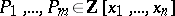have total degree at mostand coefficients of absolute value at most. For, let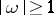be greater than or equal to the largest absolute value of the coordinates ofand letbe less than or equal to the distance fromto the common zerosof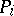. Then there are explicit constants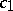,,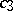depending onsuch that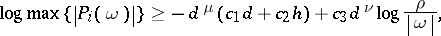where,.

Over arbitrary fields with an absolute value, the lower bound takes the form, cf. [a4], [a2] and [a1] (in the last citation, the polynomialsare replaced by ideals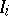andare taken to be the values of fixed Chow coordinates of). In this setting, M. Hickel [a3] obtains the optimal involvement of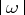at the right-hand side. Actually, the above arithmetic inequality holds with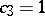.

If, when working over,denotes a zero of an unmixed idealanddenotes the distance fromto the zeros of, then the above upper bound holds with,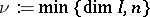, withreplaced by, and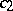by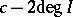. When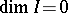, the zeros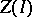ofhave algebraic coordinates. When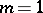and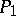does not vanish at any point of, then one obtains an explicit lower bound on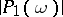, i.e. a Liouville inequality.

How to Cite This Entry:
Liouville-Łojasiewicz inequality. Encyclopedia of Mathematics. URL: http://encyclopediaofmath.org/index.php?title=Liouville-%C5%81ojasiewicz_inequality&oldid=11458
This article was adapted from an original article by W. Dale Brownawell (originator), which appeared in Encyclopedia of Mathematics - ISBN 1402006098. See original article